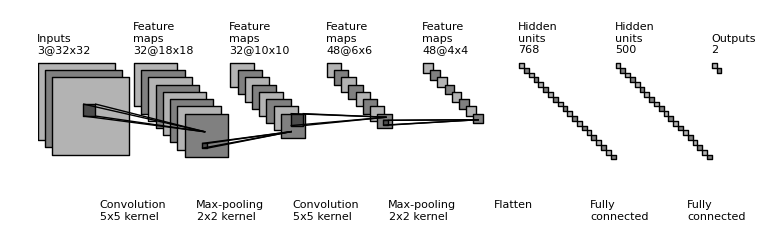This post is for cataloging those online resources that are useful to my work for the terraAI project, in particular those related to Machine Learning. Hopefully these will also be useful to other Machine Learning researchers.

##### Typesetting math formulas

It would seem that the best way to typeset formulas, which is useful when discussing topics related to Machine Learning, is to use MathJax.

Typesetting in Ghost. We will use the Bellman equation as an example below. The Bellman equation looks like this:

$V_x = \min_u \left[ r_{xu} + \gamma V_f \right]$

This equation in the Latex syntax normally looks like the following:

$V\_x = \min_u \left[ r_{xu} + \gamma V_f \right]$


Which cannot be entered into Ghost directly, since the backslashes and underscores will be consumed by the system. This can be handled by manually adding additional backslashes before those special characters, as follows:

\$V\_x = \\min\_u \\left[ r\_{xu} + \\gamma V\_f \\right]\$


Personally I prefer to use some Javascript to automate this process, so that all I have to do is to cut-and-paste the Latex code acquired from elsewhere into a pre tag as follows:

<pre class="mathjax">$V\_x = \min_u \left[ r_{xu} + \gamma V_f \right]$</pre>


The Javascript code will then scan the entire article, does the necessary processing and render it correctly.

Random samples

Following are random samples, kept here just for my own convenience as a reference. More samples can be found in the Resources by inspecting the source code for the respective webpages.

WaveNet


$p({\bf x}|{\bf h}) = \prod_{t=1}^{T}{p(x_t|x_1,...,x_{t-1}, {\bf h})}$


$\begin{equation}\tag{5五} \mathrm{z} = tanh(W_{f,k} * \mathrm{x}) \odot σ(W_{g,k} * \mathrm{x}) \end{equation}$


The skip-gram model


$\arg\max \limits_\theta \prod_{w\in Text}{\left[ \prod_{c\in C(w)} p(c|w;\theta) \right]}$

<pre class="mathjax">$\arg\max \limits_\theta \prod_{w\in Text}{\left[ \prod_{c\in C(w)} p(c|w;\theta) \right]}$


The alternative skip-gram model


$\arg\max \limits_\theta \prod_{(w,c)\in D}{ p(c|w;\theta) }$

<pre class="mathjax">$\arg\max \limits_\theta \prod_{(w,c)\in D}{ p(c|w;\theta) }$</pre>


The TAI knowledge-based "skip-gram" model


$\arg\max \limits_\theta \prod_{w\in Text}{ \left[ \prod_{c\in C(w)} \left[ \prod_{k\in K(c,w)} p(k|w;\theta) \right] \right] }$


$H_0: \mu_{A} = \mu_{B}$

\$H\_0: \mu\_{A} = \mu\_{B}\$


$f(a) = \frac{1}{2\pi i} \oint_\gamma \frac{f(z)}{z-a}, dz$

\$f(a) = \\frac{1}{2\\pi i} \\oint_\\gamma \\frac{f(z)}{z-a}, dz\$


\begin{align} Q_{xu} &= r_{xu} + \gamma V_f \\ &= r_{xu} + \gamma \min_{u'} Q_{fu'} \end{align}

\\begin{align}
Q\_{xu} &amp;= r_{xu} + \\gamma V\_f \\\\
&amp;= r\_{xu} + \\gamma \\min\_{u'} Q\_{fu'}
\\end{align}


$\hat{V}_x \leftarrow \min_u \left[ r_{xu} + \gamma \hat{V}_f \right]$

\$\hat{V}\_x \\leftarrow \\min\_u \\left[ r\_{xu} + \\gamma \\hat{V}\_f \\right]\$


$r_{xu} = Q_{xu} - \gamma \min_{u'} Q_{fu'}$

\$r\_{xu} = Q\_{xu} - \\gamma \\min\_{u'} Q\_{fu'}\$



$\hat{y} = \arg\max \limits_y P(y)\sum_{i = 1}^{M}{\log P(x_i|y)}$

<pre class="mathjax">$\hat{y} = \arg\max \limits_y P(y)\sum_{i = 1}^{M}{\log P(x_i|y)}$</pre>


$$x = {-b \pm \sqrt{b^2-4ac} \over 2a}.$$ $$x = {-b \pm \sqrt{b^2-4ac} \over 2a}.$$

$r_{xu} = Q_{xu} - \gamma \min_{u'} Q_{fu'}$

The following expression only works in this blog:

<pre class="mathjax">$r_{xu} = Q_{xu} - \gamma \min_{u'} Q_{fu'}$</pre>


Set expression, here is the inline version ${f'_i}$ and $\{f'_{i+1}\}$
\${f'\_i}\$

and following is the display version :
$$\{f' _i\}$$ $$\\{f' _i\\}$$

##### ML Code Libraries

These are useful as the code base for adding the learning capability. Candidates:

1. TensorFlow
2. Keras
3. Karpathy. Andrej dir Karpathy's various Javascript-based machine learning systems could be used as the basis for supporting in-browser machine learning, which is highly desirable for improving system scalability.
4. The OpenAI Gym toolkit
5. mxnet: for supporting deep learning on mobile devices. Blurb from literature: lightweight, Portable, Flexible Distributed/Mobile Deep Learning with Dynamic, Mutation-aware Dataflow Dep Scheduler; for Python, R, Julia, Scala, Go.
6. Brain: a JavaScript neural network library
7. Python Natural Language Toolkit. NLTK is a leading platform for building Python programs to work with human language data. It provides easy-to-use interfaces to over 50 corpora and lexical resources such as WordNet, along with a suite of text processing libraries for classification, tokenization, stemming, tagging, parsing, and semantic reasoning, wrappers for industrial-strength NLP libraries, and an active discussion forum.
8. MORE
##### ML learning resources

Following are some introductory Machine Learning material that might be useful to beginners in this area:

1. Word2vec: Neural Word Embeddings in Java by DeepLearning4j
2. MORE
##### Diagramming tools
1. Drawing a neural network - this uses JavaScript and CSS
2. D3.js : a JavaScript library for manipulating documents based on data. D3 helps you bring data to life using HTML, SVG, and CSS. D3’s emphasis on web standards gives you the full capabilities of modern browsers without tying yourself to a proprietary framework, combining powerful visualization components and a data-driven approach to DOM manipulation.
3. Python script for illustrating Convolutional Neural Network (ConvNet). Example:4. MORE
##### Resources
1. MathJax: a library for displaying math formulas, useful when discussing machine learning algorithms.
2. How to display mathematical equations in Ghost
3. Tools and examples of ML-related math formulas
4. How to insert 'LATEX' text dynamically in html
5. MORE